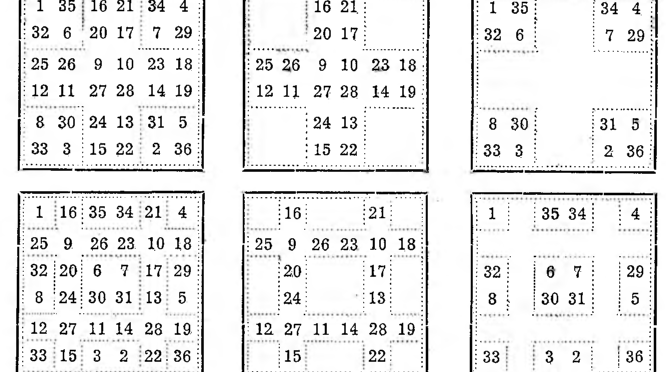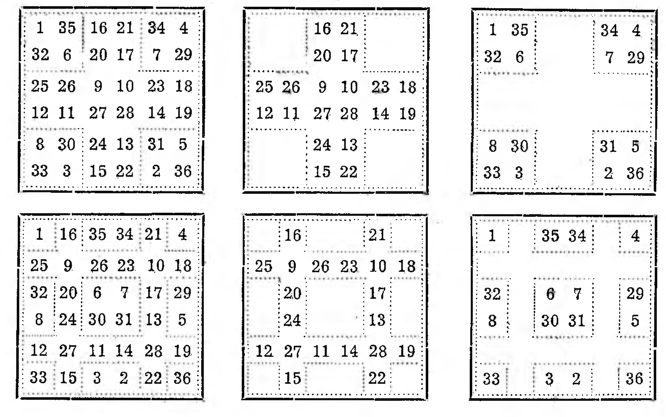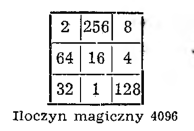# Squares with crosses

Squares with crosses. Framed squares can be easily transformed into squares with a cross or four crosses. To do this, for example, for the square 6X6, break the central square 4 X 4 into four squares 2 X 2 and we carry them to the four horns; We also divide the frame into four parts and put it in the middle in the form of a cross, taking care, so that the numbers standing at the ends of the diagonal remain on the diagonals; we will then get a magic square with a cross.
If we break the same given square into 9 parts and put them together, as shown in the picture, then we get a square with four crosses. The peculiarity of such squares lies in this, that the parts separated by the thicker line give a certain magic sum separately from the whole, namely, the crosses give a magic sum 111, a figury, which will remain after the removal of the single or quadruple cross, can be put into a square with a magic sum 74.Magic squares with geometric progress.
General rules for constructing squares from non-arithmetic progress, but geometric, are identical to the previous ones. Of course, instead of a string of numbers 1, 2, 3, 4, . . . one should use a series of exponents 0, 1, 2, 3, 4 … and instead of a magic sum you have to consider the magic product.